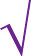# Solving Radical Equations

How to solve equations with square roots, cube roots, etc.

## Radical EquationsA Radical Equation is an equation with a square root or cube root, etc.

## Solving Radical Equations

We can get rid of a square root by squaring. (Or cube roots by cubing, etc)

But Warning: this can sometimes create "solutions" which don't actually work when we put them into the original equation. So we need to Check!

Follow these steps:

• isolate the square root on one side of the equation
• square both sides of the equation

Then continue with our solution!

### Example: solve √(2x+9) − 5 = 0

isolate the square root:√(2x+9) = 5
square both sides:2x+9 = 25

Now it should be easier to solve!

Move 9 to right: 2x = 25 − 9 = 16
Divide by 2:x = 16/2 = 8
Answer:x = 8

Check: √(2·8+9) − 5 = √(25) − 5 = 5 − 5 = 0

That one worked perfectly.

## More Than One Square Root

What if there are two or more square roots? Easy! Just repeat the process for each one.

It will take longer (lots more steps) ... but nothing too hard.

### Example: solve √(2x−5) − √(x−1) = 1

isolate one of the square roots:√(2x−5) = 1 + √(x−1)
square both sides:2x−5 = (1 + √(x−1))2

We have removed one square root.

expand right hand side:2x−5 = 1 + 2√(x−1) + (x−1)
simplify:2x−5 = 2√(x−1) + x
subtract x from both sides:x−5 = 2√(x−1)

Now do the "square root" thing again:

isolate the square root:√(x−1) = (x−5)/2
square both sides:x−1 = ((x−5)/2)2

We have now successfully removed both square roots.

Let us continue on with the solution.

Expand right hand side:x−1 = (x2 − 10x + 25)/4

It is a Quadratic Equation! So let us put it in standard form.

Multiply by 4 to remove division:4x−4 = x2 − 10x + 25
Bring all to left:4x − 4 − x2 + 10x − 25 = 0
Combine like terms:−x2 + 14x − 29 = 0
Swap all signs:x2 − 14x + 29 = 0

Using the Quadratic Formula (a=1, b=−14, c=29) gives the solutions:

2.53 and 11.47 (to 2 decimal places)

Let us check the solutions:

2.53: √(2×2.53−5) − √(2.53−1) ≈ −1 Oops! Should be plus 1.11.47: √(2×11.47−5) − √(11.47−1) ≈ 1 Yes that one works.There is really only one solution:

Answer: 11.47 (to 2 decimal places)

See? This method can sometimes produce solutions that don't really work!

The root that seemed to work, but wasn't right when we checked it, is called an "Extraneous Root"

So: Checking is important.